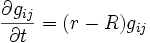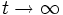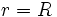# Yamabe flow

## Definition

The Yamabe flow is a flow on the space of all Riemannian metrics on a given differential manfiold. It is defined by the following differential equation for the metric$g$ as a function of$t$:$\frac{\partial g_{ij}}{\partial t} = (r-R)g_{ij}$

here$r$ is the average scalar curvature and$R$ is the scalar curvature function.

This flow preserves the conformal class of a Riemannian metric, viz the metrics at all times are the same as the initial metric in terms of conformal class.

## Facts

The motivation behind the Yamabe flow is the hope that as$t \to \infty$, we approach a constant-scalar curvature metric. If this is true, then we have positively resolved the Yamabe conjecture, which states that every conformal class of Riemannian metrics contains a constant-scalar curvature metric.

### Same as the volume-normalized Ricci flow on surfaces

On a surface, the volume-normalized Ricci flow is the same as the Yamabe flow. In fact, much of the analysis of the volume-normalized Ricci flow on surfaces has, as its correct generalization to higher dimensions, not the volume-normalized Ricci flow, but the Yamabe flow.

### Stationary points

A point is stationary for this flow if and only if it is: constant-scalar curvature metric

For a metric with constant scalar curvature$r = R$ everywhere, so the right side vanishes and hence the metric is stationary.

Note that in two dimensions, constant-scalar curvature metrics are the same as Einstein metrics, which are the fixed points under the nolume-normalized Ricci flow.